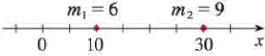Chapter 8.3, Problem 21E

Chapter
Section
Textbook Problem

Point-masses mi are located on the x-axis as shown. Find the moment M of the system about the origin and the center of mass x ¯ .21.To determine

To find: The moment (M) of the system about the origin and the center of mass (x¯).

Explanation

Given:

The mass m1=6 at a distance x1=10.

The mass m2=9 at a distance x2=30.

Number of count is n=2.

Calculation:

Calculate the moment (M) of the system about the origin:

M=i=1nmixi (1)

Substitute 2 for n in Equation (1).

M=i=12mixi=m1x1+m2x2 (2)

Substitute 6 for m1, 9 for m2, 10 for x1, and 30 for x2 in Equation (2)

Still sussing out bartleby?

Check out a sample textbook solution.

See a sample solution

The Solution to Your Study Problems

Bartleby provides explanations to thousands of textbook problems written by our experts, many with advanced degrees!

Get Started

In Exercises 41-48, find the indicated limit given that limxaf(x)=3 and limxag(x)=4 45. limxag(x)

Applied Calculus for the Managerial, Life, and Social Sciences: A Brief Approach

Find the limit or show that it does not exist. limx1+4x62x3

Single Variable Calculus: Early Transcendentals, Volume I

Solve using Cramers Rule: {2xz=143xy+5z=04x+2y+3z=2

Precalculus: Mathematics for Calculus (Standalone Book)

Solve the equations in Exercises 126. (x+1)2(2x+3)(x+1)(2x+3)2=0

Finite Mathematics and Applied Calculus (MindTap Course List)